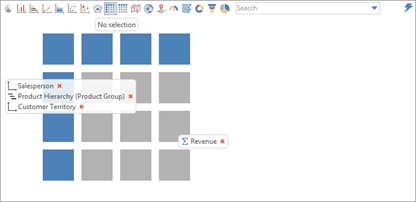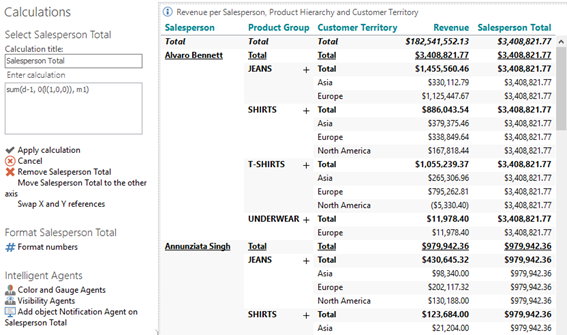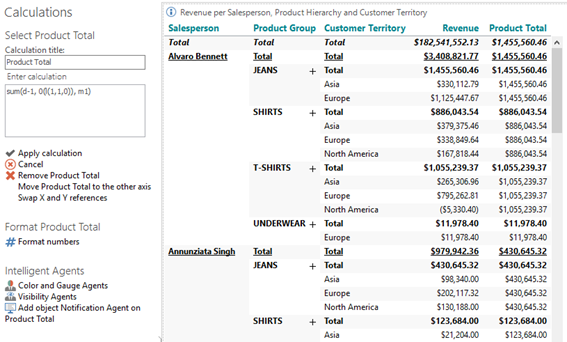# Levels with multiple dimensions on the same axis

• Add another crosstab looking at Revenue per Salesperson,  by Product Hierarchy(Product Group) and by Customer(Territory).

Now make sure that all 3 dimensions are on the same (vertical) axis as shown here:This creates a special situation in relation to the level parameter. You can actually reference the level of each dimension in one sentence.

This example references the totals of the Salespersons:

Salespersons totals: sum(d-1, 0(l(1, 0, 0)), m1)l(1, 0, 0) means level 1 on the Salesperson dimension and level 0 on Product and Customer Country (level 0 being the “all” level).

So in short – totals for Products and Customer Country but still within each Salesperson.

To reference the Product subtotals the syntax would be:

Product Subtotals = sum(d-1, 0(l(1, 1, 0)),m1)Totals on the Customer Country level – but within each Salesperson/Product Group.

### Named reference in combined heirarchy

In the above example, in case you needed to refer to a specific cell, using the “@” reference method, the syntax should be:

sum(d1, @”[Alvaro Bennett]”;”[T-SHIRTS]”;”[Europe]”, m1)

0 out of 0 found this helpful

• Hi Lara,

Your syntax, avg(d-1, 0(l(1,0,0)), m3), indicates that you are calculating the average of the current row - i.e. the average of a single cell is identical to the sum of a single cell.

If your average calculation should make sense, it should probably be one of either:

• avg(d-1, all(l(1,0,0)), m3), or
• avg(all, 0(l(1,0,0)), m3)

BR / Ole

• Hi,

Is this possible to do with the average function, rather than the sum?

I tried using the following: avg(d-1, 0(l(1,0,0)), m3)

But it returns the sum rather than the average.